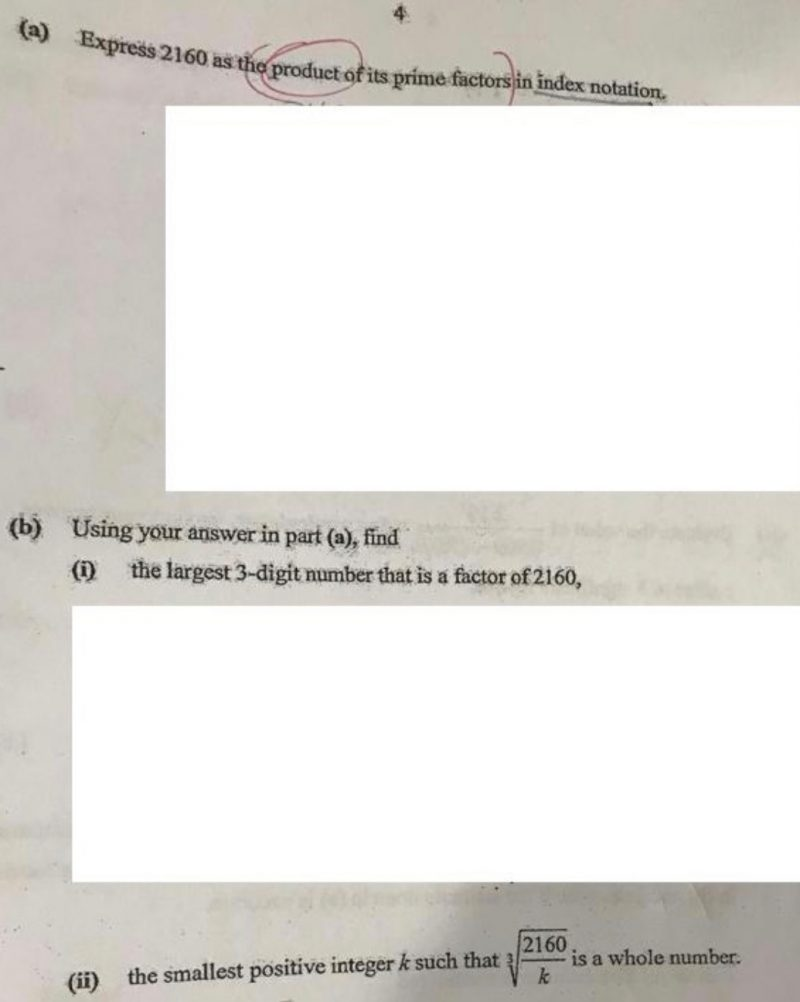Question**Dear Teachers, please help to solve part b (i) and (ii). Thanks in advance.

I think the first part is quite straight forward, you should be able to do the standard prime factorisation approach using ladder method to get 2160 = 2^4 x 3^3 x 5.

b) To get the largest 3 digit factor, you just need to divide 2160 by the smallest factor.. 2.

you will end with 540. But the question requires you to use part (a) answer, so you need to present you answers as 2^2 x 3^3 x 5

c) To get a whole number for the cube root, all the powers inside the cube root must be in multiples of three. So we need to get rid of 2 from 2^4 and 5. So K = 2 x 5  = 10.

Sorry, next time.. will take photo of my working..

hope this helps.

Pand

0 Replies 0 Likes